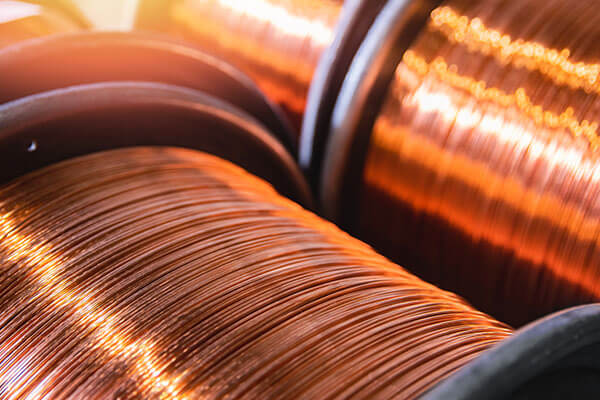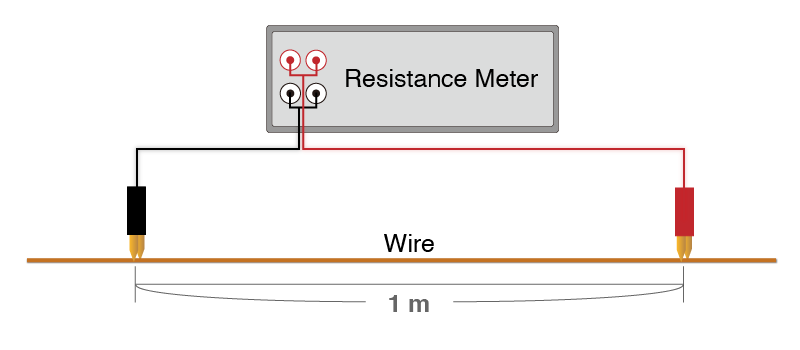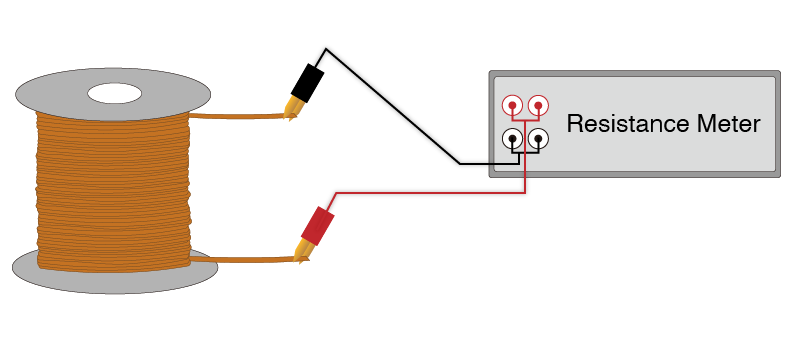# Estimating the Length of Copper Wire

## How to estimate length of wire using resistance

The length of copper wire can be verified by the following steps by using the Resistance Meter RM3544, RM3545, or RM3548.

• 1.Use the Resistance Meter RM3544, RM3545, or RM3548 to measure the resistance for one meter of the wire whose overall length (Y) you want to estimate.
• 2.Measure the resistance of the entire wire whose length (Z) you want to estimate.
• 3.Divide the resistance value of the entire wire (Z) by the resistance value of one meter (Y), to estimate the overall length of the wire (X).X [m] = Z [Ω] / Y [Ω/m]

• X [m] = Unknown length of the wire
• Y [Ω/m] = Resistance of 1 m (see Fig. 1)
• Z [Ω] = Resistance value of the entire wire (see Fig. 2)
•Fig. 1
•Fig. 2

## To ensure accurate measurement

Since copper wire has a comparatively large temperature coefficient, error will be introduced if the temperature of the wire when the resistance per meter is measured differs from the temperature of the wire when the whole resistance is measured.

The resistance meter’s temperature correction function can be used to correct this temperature variations in the wire’s resistance.